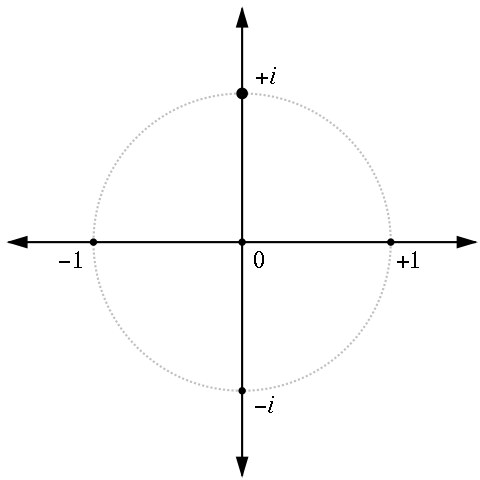# -1 = 1

Algebra Level 2I have found a proof that $-1=1$. Or have I? If you think one of these steps is wrong, input your answer as the step number.

Step 1: $-1$
Step 2: $={ i }^{ 2 }$
Step 3: $=i\times i$
Step 4: $=\sqrt { -1 } \times \sqrt { -1 }$
Step 5: $=\sqrt { -1\times -1 }$
Step 6: $=\sqrt { 1 }$
Step 7: $=1$

×